# Sample release¶

This notebook serves as a basic introduction to loading and viewing data released in associaton with the GWTC-2 Parameter Estimation Data Release.

The released data file can be read in using the h5py, PESummary, or astropy libraries*. For general instructions on how to manipulate the data file and/or read this data file with h5py, see the PESummary docs

In this notebook we use as an example the event GW190519_153544. The tar file containing the data that is used can be found here: https://dcc.ligo.org/LIGO-P2000223 . We assume the tar file is unarchived in the same directory as this notebook.

* We do not guarantee that the data release files can be read in with other packages.

First we import the key python modules

In :
%matplotlib inline
import pesummary
print(pesummary.__version__)
import h5py
%config InlineBackend.figure_format = 'retina'

0.9.1


Note that for pesummary<=0.9.1, seaborn<=0.10.1 is required.

The samples for each event is stored in the corresponding h5 file. This data file can be read either using h5py or using in using the pesummary read function. Each analysis file will contain several datasets. For a detailed description of what the names mean, see Table III and Table VIII of https://dcc.ligo.org/LIGO-P2000061.

In :
file_name = './GW190519_153544/GW190519_153544_comoving.h5'

In :
# Using h5py
with h5py.File(file_name, 'r') as f:
print('H5 datasets:')
print(list(f))

H5 datasets:
['C01:IMRPhenomD', 'C01:IMRPhenomPv2', 'C01:NRSur7dq4', 'C01:SEOBNRv4P', 'C01:SEOBNRv4PHM', 'C01:SEOBNRv4P_nonevol', 'PrecessingSpinIMR', 'PrecessingSpinIMRHM', 'PublicationSamples', 'ZeroSpinIMR', 'history', 'version']

In :
# Using pesummary
print('Found run labels:')
print(data.labels)

/Users/sergei/anaconda3/envs/v4PHM/lib/python3.6/site-packages/statsmodels/tools/_testing.py:19: FutureWarning: pandas.util.testing is deprecated. Use the functions in the public API at pandas.testing instead.
import pandas.util.testing as tm

Found run labels:
['C01:IMRPhenomD', 'C01:IMRPhenomPv2', 'C01:NRSur7dq4', 'C01:SEOBNRv4P', 'C01:SEOBNRv4PHM', 'C01:SEOBNRv4P_nonevol', 'PrecessingSpinIMR', 'PrecessingSpinIMRHM', 'PublicationSamples', 'ZeroSpinIMR']


For the remainder of the notebook, we demonstrate how to use pesummary to access and plot various aspects of the analysis.

The posterior samples can be extracted through the samples_dict property. These posterior samples are stored in a custom table structure. Below we load a particular dataset and show which parameters are available. For a detailed description of the meaning of most parameters, see definition of standard parameters

In :
samples_dict = data.samples_dict
posterior_samples = samples_dict['PrecessingSpinIMRHM']
parameters = sorted(list(posterior_samples.keys()))
print(parameters)

['Npts', 'a_1', 'a_2', 'chi_eff', 'chi_p', 'chirp_mass', 'chirp_mass_source', 'comoving_distance', 'cos_iota', 'cos_theta_jn', 'cos_tilt_1', 'cos_tilt_2', 'dec', 'final_mass', 'final_mass_non_evolved', 'final_mass_source', 'final_mass_source_non_evolved', 'final_spin', 'final_spin_non_evolved', 'geocent_time', 'inverted_mass_ratio', 'iota', 'log_likelihood', 'luminosity_distance', 'mass_1', 'mass_1_source', 'mass_2', 'mass_2_source', 'mass_ratio', 'neff', 'p', 'peak_luminosity', 'peak_luminosity_non_evolved', 'phase', 'phi_1', 'phi_12', 'phi_2', 'phi_jl', 'ps', 'psi', 'psiJ', 'ra', 'radiated_energy', 'radiated_energy_non_evolved', 'redshift', 'spin_1x', 'spin_1y', 'spin_1z', 'spin_2x', 'spin_2y', 'spin_2z', 'symmetric_mass_ratio', 'theta_jn', 'tilt_1', 'tilt_2', 'total_mass', 'total_mass_source']


## PrecessingSpinIMRHM analysis¶

pesummary allows for the user to easily make plots. As an example, we show the posterior distribution for chirp_mass_source plotted as a histogram and as a KDE.

In :
fig = posterior_samples.plot('chirp_mass_source', type='hist')
posterior_samples.plot('chirp_mass_source', type='hist',fig=fig, kde=True)
fig.show()

/Users/sergei/anaconda3/envs/v4PHM/lib/python3.6/site-packages/ipykernel_launcher.py:3: UserWarning: Matplotlib is currently using module://ipykernel.pylab.backend_inline, which is a non-GUI backend, so cannot show the figure.
This is separate from the ipykernel package so we can avoid doing imports until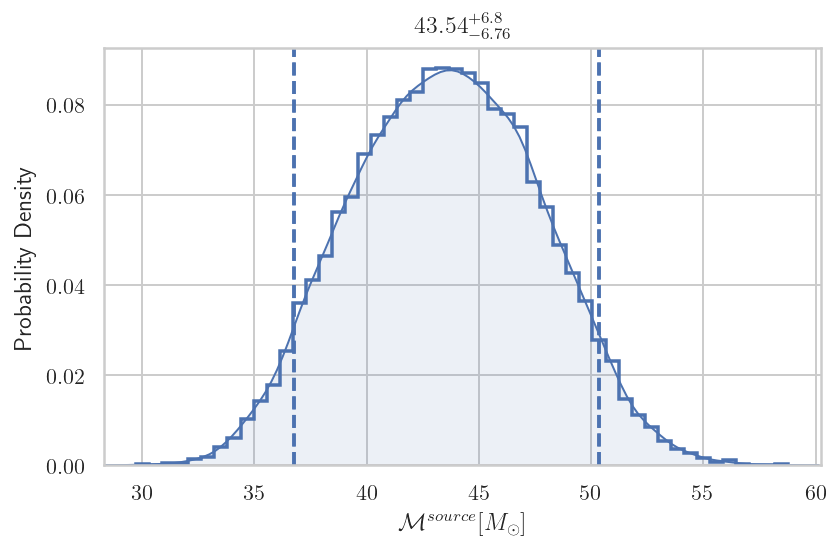We may also easily generate a spin disk, showing the most probable direction of the spin vectors

In :
fig = posterior_samples.plot(type='spin_disk', colorbar=True, annotate=True,
show_label=True, cmap='Blues')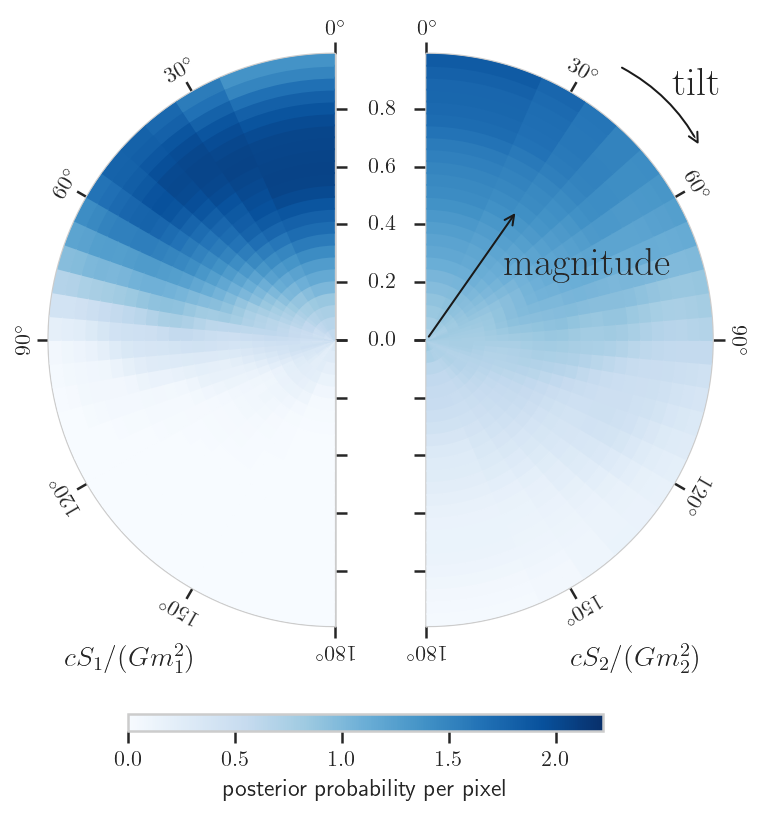Corner plots are very useful for spotting degeneracies between parameters. A corner plot can easily be generated using 'pesummary'

In :
fig = posterior_samples.plot(type='corner',
parameters=['mass_1', 'mass_2', 'luminosity_distance', 'iota'])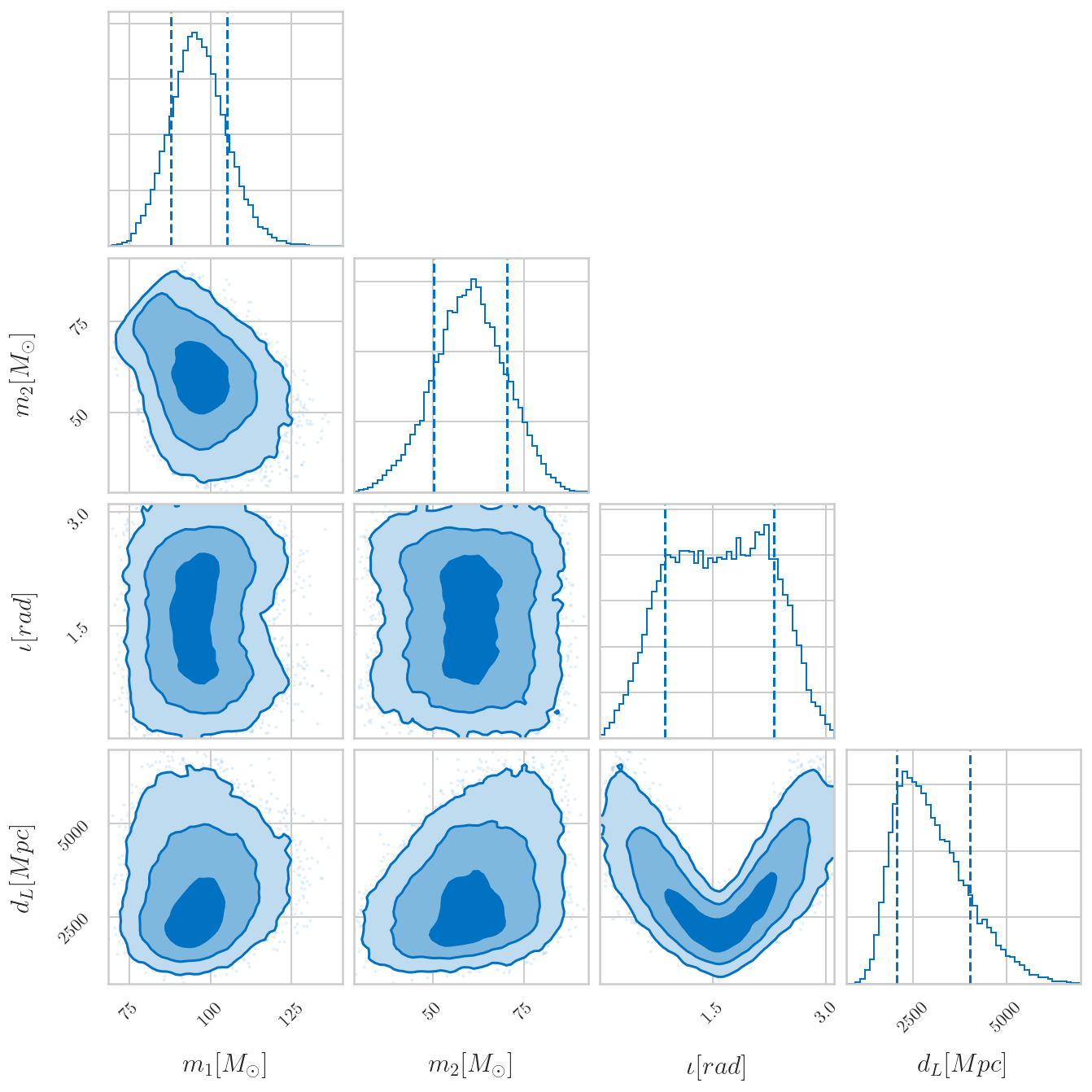## Comparing multiple analyses¶

In this example, we compare results from 3 diffrent waveforms: IMRPhenomPv2,SEOBNRv4Pand NRSur7dq4.

In :
# Set a consistent color scheme
cp = ['#1b9e77','#d95f02','#7570b3']

In :
labels_of_interest = ['C01:IMRPhenomPv2','C01:SEOBNRv4P','C01:NRSur7dq4']
fig=samples_dict.plot('chirp_mass', type='hist', kde=True,labels=labels_of_interest,colors=cp)
fig.show()

/Users/sergei/anaconda3/envs/v4PHM/lib/python3.6/site-packages/ipykernel_launcher.py:3: UserWarning: Matplotlib is currently using module://ipykernel.pylab.backend_inline, which is a non-GUI backend, so cannot show the figure.
This is separate from the ipykernel package so we can avoid doing imports until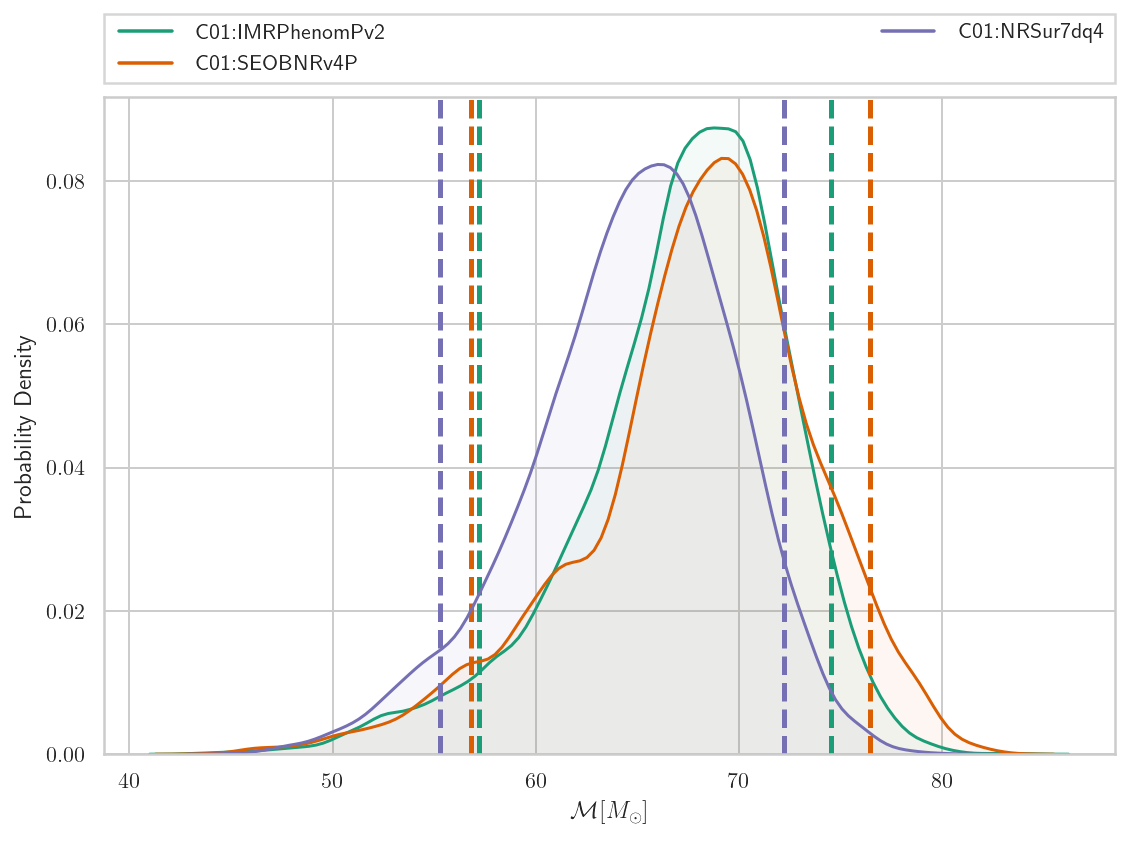A comparison histogram is not the only way to display this data. We may also generate a violin plot showing the posterior distribution for each analysis

In :
fig = samples_dict.plot('chirp_mass', type='violin',labels=labels_of_interest,palette=cp)
fig.show()

/Users/sergei/anaconda3/envs/v4PHM/lib/python3.6/site-packages/ipykernel_launcher.py:2: UserWarning: Matplotlib is currently using module://ipykernel.pylab.backend_inline, which is a non-GUI backend, so cannot show the figure.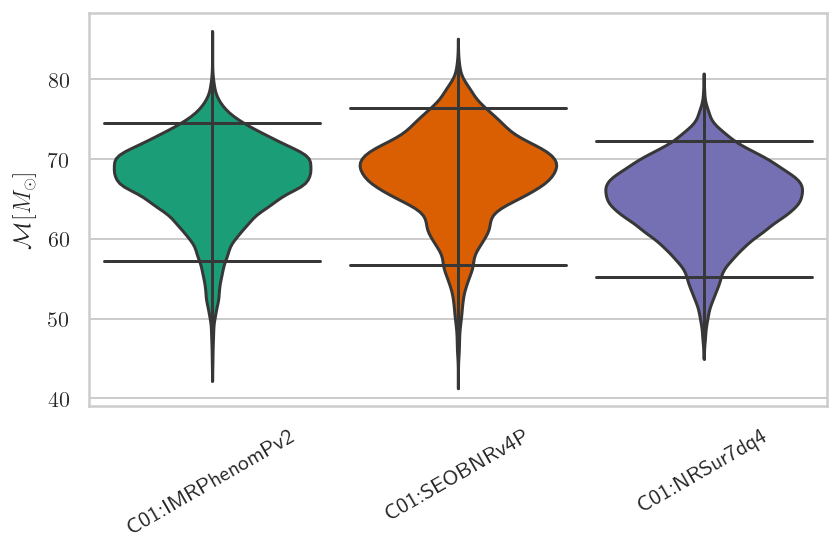Here is an example of generating a triangle plot:

In :
fig = samples_dict.plot(['mass_1', 'mass_2'], type='reverse_triangle',
grid=False,labels=labels_of_interest,colors=cp)
fig.show()

/Users/sergei/anaconda3/envs/v4PHM/lib/python3.6/site-packages/ipykernel_launcher.py:3: UserWarning: Matplotlib is currently using module://ipykernel.pylab.backend_inline, which is a non-GUI backend, so cannot show the figure.
This is separate from the ipykernel package so we can avoid doing imports until